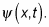##### Quantum Physics For Dummies, Revised EditionThere are plenty of free particles — particles outside any square well —in the universe, and quantum physics has something to say about them. The discussion starts with the Schrödinger equation: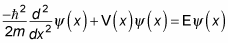Say you’re dealing with a free particle whose general potential, V(x) = 0. In that case, you’d have the following equation: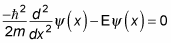And you can rewrite this as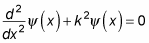where the wave number, k, is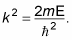You can write the general solution to this Schrödinger equation as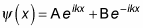If you add time-dependence to the equation, you get this time-dependent wave function: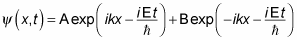That’s a solution to the Schrödinger equation, but it turns out to be unphysical. To see this, note that for either term in the equation, you can’t normalize the probability density,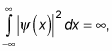as long as A and B aren’t both equal to zero.

What’s going on here? The probability density for the position of the particle is uniform throughout all x! In other words, you can’t pin down the particle at all.

This is a result of the form of the time-dependent wave function, which uses an exact value for the wave number,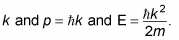So what that equation says is that you know E and p exactly. And if you know p and E exactly, that causes a large uncertainty in x and t — in fact, x and t are completely uncertain. That doesn’t correspond to physical reality.

For that matter, the wave function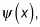Marylouise, can you format the EQ above as a gif? Thanks, Alexa.

as it stands, isn’t something you can normalize. Trying to normalize the first term, for example, gives you this integral:EQ needs to be a gif.

Remember that the asterisk symbol (*) means the complex conjugate. A complex conjugate flips the sign connecting the real and imaginary parts of a complex number.

And for the first term of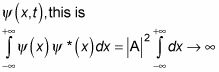EQ needs to be a gif.

And the same is true of the second term in